Smartick is an advanced online program that teaches kids math and coding in only 15 min. a day

Feb17

# Combining Decimals and Fractions

Imagine that you have to solve the following addition problem that combines decimals and fractions: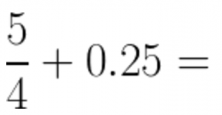It’s not so easy to add a fraction to a decimal, right? It’s much easier to add fractions to fractions, or decimals to decimals. So, we can do this in two ways:

• Change the decimal to a fraction.
• Change the fraction to a decimal.

Today, we are going to learn how to do the first option: change decimals into fractions. In order to begin, you first need to know how to find equivalent fractions.

In any case, in this post we’re going to do a quick review on finding equivalent fractions:

### Finding Equivalent Fractions

Example: Here is our fraction…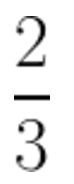And we want to make its denominator the number 6, or in other words: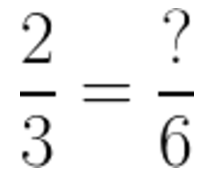You have to ask yourself, what number can 3 (the denominator) be multiplied by in order to get 6…that’s it! It was multiplied by 2.

So, the numerator has to be multiplied by 2 as well.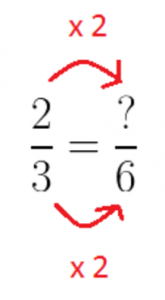If we multiply 2 by 2, we get 4.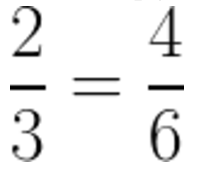Okay, now that you know how to find an equivalent fraction, we’re going to change a decimal into a fraction.

### Changing a Decimal to a Fraction

Suppose we have the number…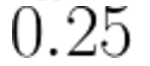How can we change it to a fraction? We’re going to follow this strategy here, but first, we need to ask ourselves what denominator the number has…what denominator can any number have that would allow it to stay the same?… That’s it! The number 1.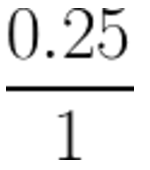Now, we have to ask ourselves, what number do we need to put in the denominator of the equivalent fraction? The trick is to use the 1 and put zeros after it. So, the first thing we’ll do is try it out with one zero, 10.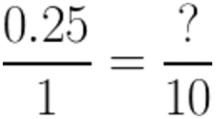Since we have to multiply 1 by 10 in order to get 10, we have to multiply 0.25 (the numerator) by 10 as well.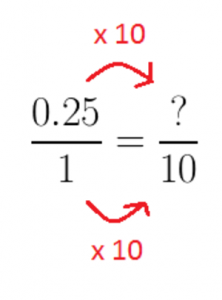And we’re left with….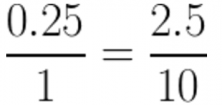We haven’t gotten rid of all the decimals yet, right? Then, let’s keep adding zeros!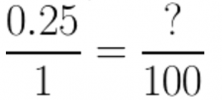If we multiply by 100, we’re left with: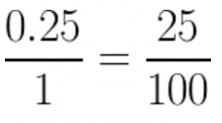Alright, remember that fractions can be simplified. If we simplify this fraction, we’re left with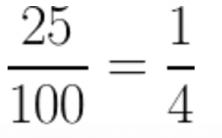So…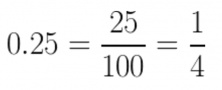And that’s it! We’ve converted a decimal to a fraction, all thanks to equivalent fractions.

### Review

Think about it, how many zeros did we have to add after the 1 in order to get rid of all the decimals in 0.25? It had two decimals and we added two zeros to it, or in words, one zero for every decimal that it had. So then, every zero cancels out one decimal!

To sum up, when we want to change a decimal into a fraction, we have to:

• Change the decimal into a fraction over 1. So, the decimal would be the numerator, and the 1 the denominator.
• Find the equivalent fraction. The denominator of this fraction will have 1 followed by as many zeros as there are decimals in the number being converted.
• Multiply the decimal by the denominator of the equivalent fraction.
• Simply the fraction.

Now, let’s go back to the problem we saw at the beginning of this post!Then,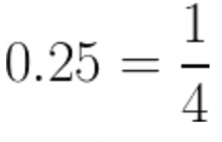Next,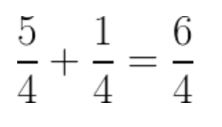We now simplify,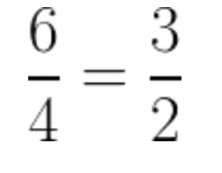Now the problem is solved!

I’ll leave you with some resources that you can use to review and practice:

And at Smartick, you’ll find plenty more exercises.